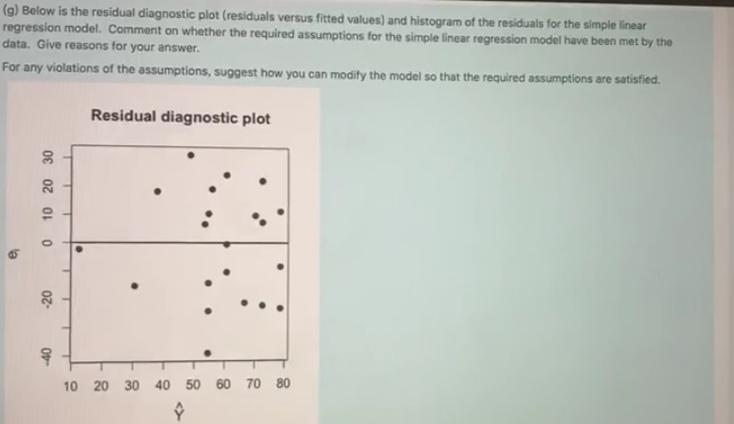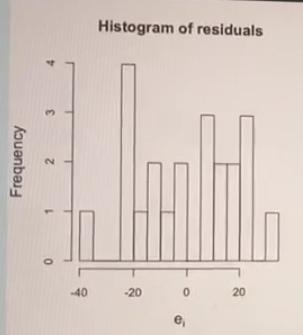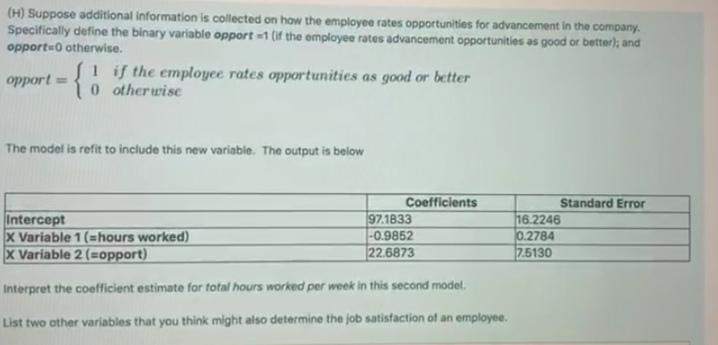# Statistics linear regression line

Question 3

Are employees who work more hours per week less satisfied with their job? Data was collected from a random sample of 22 employees at a company on the following variables:

• Job satisfaction (measures 0 to 100)
• Total hours worked per week
 Coefficients Standard Error intercept 120.7223 16.8721 X variable 1 -1.2033 0.3188
1. which variable is the independent (explanatory) variable for this simple linear regression model? Which variable is the (response) dependent variable?

(b)State the simple linear regression equation

(c)Interpret the meaning of the slope coefficient estimate in this problem

(d)at the 0.05 level of significance, is there evidence of a linear relationship between total hours and job satisfaction? Provide a reason for your answer.

(e)the ANOVA table of the regression model fit is shown below (with some items missing)

 df SS MS Significance F Regression ?? ?? 142419 0.0012 Residual 20 8074.6720 403.7340 Total 21 13824.591

What proportion of the variation in the response variable can be explained by the variation in the explanatory variable in the simple linear regression model? Please show your working to support your answer

(f) construct a 95% confidence interval estimate of the population mean job satisfaction for employees who works 55 hours per week. The sample mean total hours worked per week was 51.1818 and the sample standard deviation was 13.7517. please show your working to support your answer.

(g)Below is the residual diagnostic plot (residual versus fitted values) and histogram of the residuals for the simple linear regression model. Comment on whether the required assumptions for the simple linear regression model have met by the data. Give reasons for your answer.

For any violations of the assumptions, suggest how you can modify the model so that the required assumptions are satisfied(H)(i)Suppose the 3 additional specified in part (H) and the variable hours worked are included in a multiple linear regression model to predict job satisfaction. The Coefficient of determination (R2)is calculated and found to be higher than R2 value for the simple linear regression model fit in part (a). Does this mean should automatically choose the bigger model with 4 explanatory variables? Explain your answer